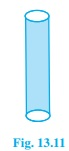# A metal pipe is 77 cm long. The inner diameter of a cross section is 4 cm, the outer diameter being 4.4cm. (see fig.) . Find its (i) inner curved surface area, (ii) outer curved surface area (iii) total surface area (Assume π = 22/7)Given

Height of Cylindrical metal pipe (h) =77 cm

The inner diameter of metal pipe (d)=4cm,

The outer diameter of metal pipe (D)=4.4cm,

Find out

We have to find

(i) inner curved surface area,

(ii) outer curved surface area

(iii) total surface area

Solution:

The inner diameter of metal pipe (d)=4cm,

The outer diameter of metal pipe (D)=4.4cm,

Inner curved surface area=2πrh =2*(22/7)*2*77

=968 cm2

Outer curved surface area=2πrh =2*(22/7)*2.2*77

=1064.8 cm2

Total Surface Area=Inner curved surface area+Outer curved surface area+area of two bases

As pipe is hollow, area of two bases is a ring area given by=2(πR2-πr2) = 2π(R2-r2)

=968+1064.8+2×(22/7)×(2.22-22)

= 2031.8+5.28

= 2038.08 cm2

Hence, Total surface area =2038.08 cm2